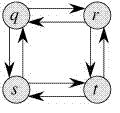Optimal Substructure Property in Dynamic Programming | DP-2

• Difficulty Level : Easy
• Last Updated : 18 Jul, 2021

As we discussed in Set 1, following are the two main properties of a problem that suggest that the given problem can be solved using Dynamic programming:
1) Overlapping Subproblems
2) Optimal Substructure

We have already discussed Overlapping Subproblem property in the Set 1. Let us discuss Optimal Substructure property here.

2) Optimal Substructure: A given problems has Optimal Substructure Property if optimal solution of the given problem can be obtained by using optimal solutions of its subproblems.

For example, the Shortest Path problem has following optimal substructure property:
If a node x lies in the shortest path from a source node u to destination node v then the shortest path from u to v is combination of shortest path from u to x and shortest path from x to v. The standard All Pair Shortest Path algorithm like Floyd–Warshall and Single Source Shortest path algorithm for negative weight edges like Bellman–Ford are typical examples of Dynamic Programming.

On the other hand, the Longest Path problem doesn’t have the Optimal Substructure property. Here by Longest Path we mean longest simple path (path without cycle) between two nodes. Consider the following unweighted graph given in the CLRS book. There are two longest paths from q to t: q→r→t and q→s→t. Unlike shortest paths, these longest paths do not have the optimal substructure property. For example, the longest path q→r→t is not a combination of longest path from q to r and longest path from r to t, because the longest path from q to r is q→s→t→r and the longest path from r to t is r→q→s→t.We will be covering some example problems in future posts on Dynamic Programming